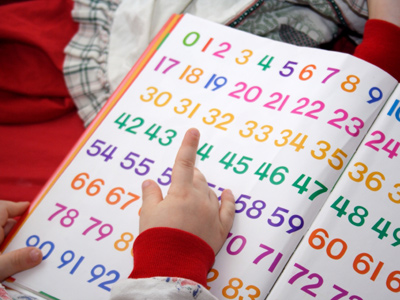Number lines are a useful tool when doing additions or subtractions.

# Numbers on a Number Line - Counting on from any Given Number

This Math quiz is called 'Numbers on a Number Line - Counting on from any Given Number' and it has been written by teachers to help you if you are studying the subject at elementary school. Playing educational quizzes is a fun way to learn if you are in the 1st or 2nd grade - aged 6 to 8.

It costs only \$12.50 per month to play this quiz and over 3,500 others that help you with your school work. You can subscribe on the page at Join Us

By their second year of elementary school children should be quite familiar with the number line. They may use it to count on or back, or to calculate additions or subtractions. Understanding the value of numbers means being able to recognize where numbers should come in relation to each other, as well as being able to recognize any missing numbers in a set.

This quiz will help children to use number lines when counting on from any given number.

Can you place the numbers on the number line?

Question 1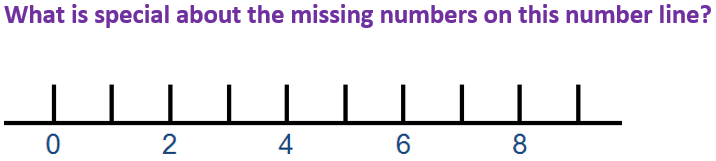They are all even
They are all odd
They are all over 10
They all end in 5
The missing numbers are 1, 3, 5, 7 and 9, which are all odd numbers
Question 2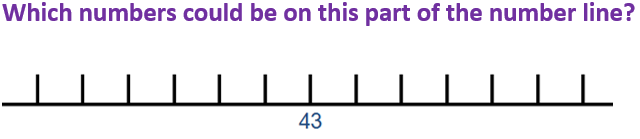39, 44, 47
8, 9, 10
77, 87, 90
20, 23, 27
Counting backwards and forwards from 43 includes the numbers 39, 44 and 47
Question 3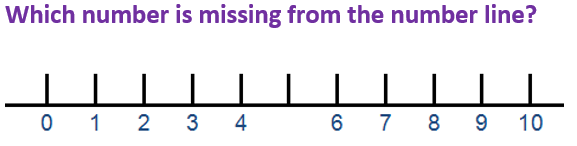5
8
9
2
4 comes before 5, 6 comes after
Question 4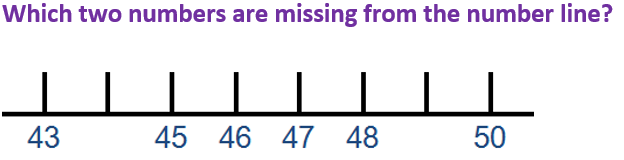44 and 51
45 and 49
51 and 58
44 and 49
Looking at the numbers on either side might be helpful
Question 5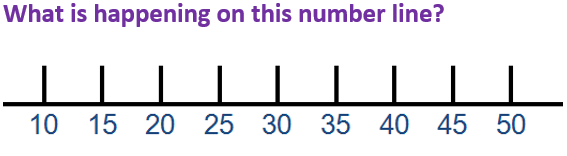The numbers are getting smaller
The numbers are all even
The numbers are going up in 5s
The numbers are all odd
Each number is 5 more than the one before it
Question 6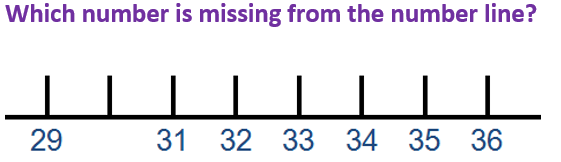25
30
39
28
30 comes before 31, and after 29
Question 7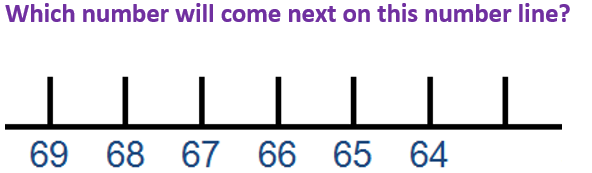60
67
63
70
The numbers are going down, not up
Question 8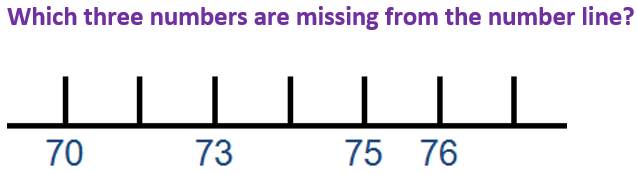75, 76 and 77
71, 74 and 77
74, 75 and 79
80, 90 and 100
Counting up aloud will help identify the missing numbers
Question 9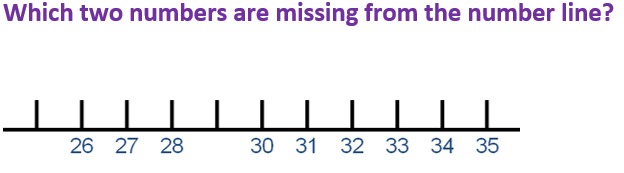26 and 31
25 and 29
29 and 40
23 and 27
25 comes before 26, and 29 comes between 28 and 30
Question 10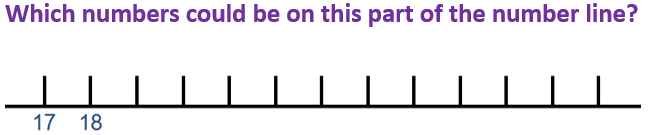88, 89, 90
2, 3, 4
66, 67, 68
20, 21, 22
Counting up from 17 and 18 would include 20, 21 and 22
Author:  Angela Smith